Worksheet: Linear Equations in Two Variables

# Worksheet: Linear Equations in Two Variables - Mathematics (Maths) Class 9

 Table of contentsWorksheet- 1Worksheet- 2Worksheet- 3Worksheet- 4Worksheet- 5## Worksheet- 1

1. Write each of the following as an equation in two variables:
(i) x = –3
(ii)y = 2
(iii) 2x = 3
(iv) 2y = 5

2. Write each of the following equations in the form ax + by + c = 0 and also write the values of a, b and c in each case:
(i) 2x + 3y = 3.47
(ii) x – 9 = √3 y
(iii) 4 = 5x – 8y
(iv) y = 2x

1. (i) x + (0)y + 3 = 0
(ii) (0)x + y = 2
(iii) 2x + (0)y – 3 = 0
(iv) (0)x + 2y – 5 = 0

2. (i) 2x + 3y – 3.47 = 0; a = 2, b = 3 and c = –3.47
(ii) x – √3y – 9 = 0; a = 1, b = - √3 and c = –9
(iii) –5x + 8y + 4 = 0; a = –5, b = 8 and c = 4
(iv) –2x + y + 0 = 0; a = –2, b = 1 and c = 0

## Worksheet- 2

1. (a) Is (3, 2) a solution of 2x + 3y = 12?
(b) Is (1, 4) a solution of 2x + 3y = 12?
(c) Is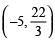a solution of 2x + 3y = 12?
(d) Is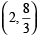a solution of 2x + 3y = 12?
2. Find four different solutions of the equation x + 2y = 6.

3. Find two solutions for each of the following equations:
(i) 4x + 3y = 12
(ii) 2x + 5y = 0
(iii) x + 3y + 4 = 0
4. Find the value of k such that x = 2 and y = 1 is a solution of the linear equation 2x – ky + 7 = 8

1. (a)Yes
(b)No
(c)Yes
(d)Yes

2. (i) (2, 2)
(ii) (0, 3)
(iii) (6, 0)
(iv) (4, 1)

3. (i) (0, 4) and (3, 0)
(ii) (0, 0) and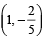(iii)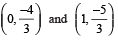## Worksheet- 3

1. Write a linear equation such that the point (1, 2) lies on its graph.

2. Draw the graph of x + y = 9.

3. Force applied on a body is directly proportional to the acceleration produced in the body.
Write an equation to express this situation and plot the graph of the equation.

4. For each of the graph given in the following figure select the equation whose graph it is from the choices given below:
Fig. (a)
(i) x + y = 0
(ii) x – y = 0
(iii) 2x = y
(iv) y = 2x + 1

Fig. (b)
(i) x + y = 0
(ii) x – y = 0
(iii) y = 2x + 4
(iv) y = x – 4

Fig. (c)
(i) x + y = 0
(ii) x – y = 0
(iii) y = 2x + 1
(iv) y = 2x – 4

Fig. (d)
(i) x + y = 0
(ii) x – y = 0
(iii) 2x + y = –4
(iv) 2x + y = 4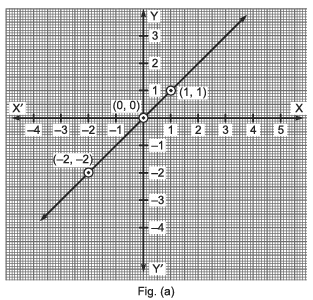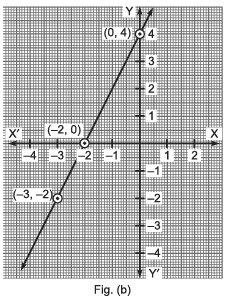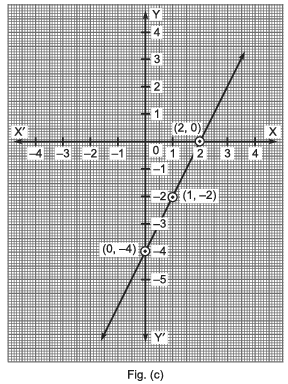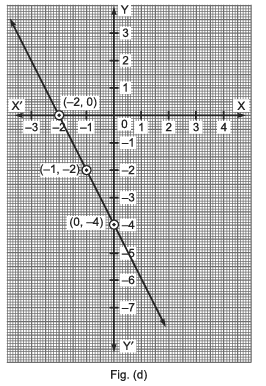1. x + y = 3

3. y = 3x

4. (a) x – y = 0
(b) y = 2x + 4
(c) y = 2x – 4
(d) 2x + y = –4

## Worksheet- 4

1. Give the geometric representation of x = 7 as an equation
(i) in one variable
(ii) in two variables
2. Solve the equation 3x + 1 = 2x – 1 and represent the solution(s) on:
(i) a number line
(ii) the cartesian plane
Hint: (ii) 3x + 1 = 2x – 1 ⇒ 3x – 2x = –1 – 1 ⇒ x = –2 ⇒ x + 0y = –2

## Worksheet- 5

1. Which of the following is not a linear equation in two variables?
(i) px + qy + c = 0
(ii) ax2 + bx + c = 0
(iii) 3x + 2y = 5
2. One of the solutions of the linear equation 4x – 3y + 6 = 0 is
(i) (3, 2)
(ii) (–3, 2)
(iii) (–3, –2)
3. lx + my + c = 0 is a linear equation in x and y. For which of the following, the ordered pair (p, q) satisfies it:
(i) lp + mq + c = 0
(ii) y = 0
(iii) x + y = 0
(iv) x = y
4. What is the equation of the x-axis?

5. What is the equation of the y-axis?

6. How many solutions do a linear equation in two variables x and y have?

7. How many solutions do the equation 5x + 2y = 7 can have?

8. Which of the following can be a form of solution of 3x + 0y + 7 = 0 in two variables?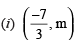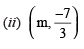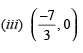9. What is the form of any point on the line y = x?

10. At which point the linear equation 2x + 3y = 6 cuts the y-axis?

11. Write the point where the graph of linear equation 2x + 9y = 8 cuts the x-axis.

12. What is the general form of a point on the y-axis?

13. What is the general form of a point on the x-axis?

14. Write the situation of the graph of y = 9 on the cartesian axis.

15. Write the linear equation whose solution is x = –1, y = 1?

16. The point (m, m) always lies on which of the following lines?
x – y = 0    or    x + y = 2m

17. How many linear equations in x and y can have a solution as (x = 1, y = 3)?

18. Through which of the following points, the graph of y = – x passes?
(i) (1, 1)
(ii) (0, 1)
(iii) (–1, 1)

19. Through which of the following points, the graph of the linear equation 3x – 2y = 0, passes?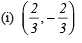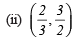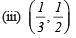20. On which of the following equations, the point of the form (m, –m) lies?
(i) x = –m
(ii) x + y = 0
(iii) y = x

21. Do the points (2, 0), (–3, 0), (0, 2), (0, –5) lie either on x-axis or y-axis?

22. Which equation’s graph is at a distance 3 units to the left of y-axis? x = 3; x = –3; or y = x

23. The graph of which of the following equations passes through the origin?
(i) y = 2x + c
(ii) y = 2x – c
(iii) y = 2x

24. Fill in the blank: The equation 2x + 5y = 7 has a unique solution if x and y are ......

1. ax2 + bx + c = 0

2. –3, –2

3. lp + mq + c = 0

4. y = 0

5. x = 0

6. infinitely many solutions

7. infinitely many solutions

8.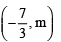9. (a, a)

10. (0, 2)

11. (4, 0)

12. (0, y)

13. (0, x)

14. Parallel to x-axis at a distance 9 units from the origin.

15. x + y = 0

16. the line x – y = 0

17. infinitely many equations in x and y 18. (–1, 1)

19.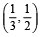20. x + y = 0

21. neither x-axis nor y-axis

22. x = –3

23. y = 2x

24. Natural numbers

The document Worksheet: Linear Equations in Two Variables | Mathematics (Maths) Class 9 is a part of the Class 9 Course Mathematics (Maths) Class 9.
All you need of Class 9 at this link: Class 9

## Mathematics (Maths) Class 9

42 videos|378 docs|65 tests

## FAQs on Worksheet: Linear Equations in Two Variables - Mathematics (Maths) Class 9

 1. What are linear equations in two variables?Ans. Linear equations in two variables are equations that represent a straight line on a coordinate plane. They involve two variables, usually represented as x and y, and can be written in the form ax + by = c, where a, b, and c are constants.
 2. How do you solve a linear equation in two variables?Ans. To solve a linear equation in two variables, we need to find the values of both variables that satisfy the equation. This can be done by substituting the given values of one variable into the equation and solving for the other variable. Once we have the value of one variable, we can substitute it back into the equation to find the value of the other variable.
 3. What is the importance of linear equations in two variables in real life?Ans. Linear equations in two variables are used in various real-life scenarios, such as determining the cost of a product based on the number of units sold, calculating the trajectory of a projectile, or analyzing the relationship between two quantities. They provide a mathematical model to solve problems and make predictions in fields like economics, physics, engineering, and more.
 4. Can linear equations in two variables have more than one solution?Ans. Yes, linear equations in two variables can have more than one solution. This occurs when the equation represents a line that intersects the coordinate plane at multiple points. In such cases, any point on the line will satisfy the equation, resulting in infinitely many solutions.
 5. How can linear equations in two variables be graphically represented?Ans. Linear equations in two variables can be graphically represented by plotting the points that satisfy the equation on a coordinate plane and connecting them to form a straight line. The slope-intercept form, y = mx + b, where m represents the slope and b represents the y-intercept, is often used to graphically represent linear equations. The slope determines the steepness of the line, while the y-intercept represents the point where the line intersects the y-axis.

## Mathematics (Maths) Class 9

42 videos|378 docs|65 testsExplore Courses for Class 9 examSignup to see your scores go up within 7 days! Learn & Practice with 1000+ FREE Notes, Videos & Tests.
10M+ students study on EduRev
Track your progress, build streaks, highlight & save important lessons and more!
Related Searches

,

,

,

,

,

,

,

,

,

,

,

,

,

,

,

,

,

,

,

,

,

;